Courses

# RS Aggarwal Test: Quadratic Equations

## 10 Questions MCQ Test Mathematics (Maths) Class 10 | RS Aggarwal Test: Quadratic Equations

Description
This mock test of RS Aggarwal Test: Quadratic Equations for Class 10 helps you for every Class 10 entrance exam. This contains 10 Multiple Choice Questions for Class 10 RS Aggarwal Test: Quadratic Equations (mcq) to study with solutions a complete question bank. The solved questions answers in this RS Aggarwal Test: Quadratic Equations quiz give you a good mix of easy questions and tough questions. Class 10 students definitely take this RS Aggarwal Test: Quadratic Equations exercise for a better result in the exam. You can find other RS Aggarwal Test: Quadratic Equations extra questions, long questions & short questions for Class 10 on EduRev as well by searching above.
QUESTION: 1

### Which of the following is not a quadratic equation?

Solution: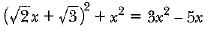2x+ 3 + 2√6x + x2 = 3x2 - 5x
3 + 2√6x + 5x= 0

Which is not a quadratic equation.

QUESTION: 2

### Which of the following equations has no real roots ?

Solution:

(a) The given equation is x2 - 4x + 3√2 = 0.
On comparing with ax2 + bx + c = 0, we get
a = 1, b = -4 and c = 3√2
The discriminant of x2 - 4x + 3√2 = 0 is
D = b2 - 4ac
= (-4)2 - 4(1)(3√2) = 16 - 12√2 = 16 - 12 x (1.41)
= 16 - 16.92 = -0.92
⇒ b2 - 4ac < 0
(b) The given equation is x2 + 4x - 3√2 = 0
On comparing the equation with ax2 + bx + c = 0, we get
a = 1, b = 4 and c = -3√2
Then, D = b2 - 4ac = (-4)2 - 4(1)(-3√2)
= 16 + 12√2 > 0
Hence, the equation has real roots.
(c) Given equation is x2 - 4x - 3√2 = 0
On comparing the equation with ax2 + bx + c = 0, we get
a = 1, b = -4 and c = -3√2
Then, D = b2 - 4ac = (-4)2 - 4(1) (-3√2)
= 16 + 12√2 > 0
Hence, the equation has real roots.
(d) Given equation is 3x​2 + 4√3x + 4 = 0.
On comparing the equation with ax2 + bx + c = 0, we get
a = 3, b = 4√3 and c = 4
Then, D = b2 - 4ac = (4√3)2 - 4(3)(4) = 48 - 48 = 0
Hence, the equation has real roots.
Hence, x2 - 4x + 3√2 = 0 has no real roots.

QUESTION: 3

### Which of the following equations has the sum of its roots as 3?

Solution:

(a) Given that, 2x2 - 3x + 6 = 0
On comparing with ax2 + bx + c = 0, we get
a = 2,  b = -3 and c = 6
∴ Sum of the roots =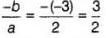So, sum of the roots of the quadratic equation 2x2 - 3x + = 0 is not 3, so it is not the answer
(b) Given that, -x2 + 3x - 3 =0
On compare with ax2 + bx + c = 0, we get
a = -1, b = 3 and c = -3
∴ Sum of the roots =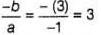So, sum of the roots of the quadratic equation -x2 + 3x - 3 = 0 is 3, so it is the answer.
(c) Given that,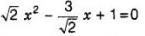⇒ 2x2 - 3x + √2 = 0
On comparing with ax2 + bx + c = 0, we get
a = 2, b = -3 and c = √2
∴ Sum of thee roots =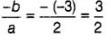So, sum of the roots of the quadratic equation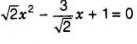is not 3, so it is not the answer.
(d) Given that, 3x2 - 3x + 3 = 0
⇒ x2 - x + 1 = 0
On comparing with ax2 + bx + c = 0, we get
a = 1, b = -1 and c = 1
∴ Sum of the roots =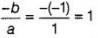So, sum of the roots of the quadratic equation 3x2 - 3x + 3 = 0 is not 3, so it is not the answer.

QUESTION: 4

(x2 + 1)2 - x2 = 0 has

Solution:

Given equation is (x2 + 1)2 - x2 = 0
⇒ x4 + 1 + 2x2 - x2 = 0 [∵ (a + b)2 = a2 + b2 + 2ab]
⇒ x4 + x2 + 1 = 0
Let x2 = y
∴ (x2)2 + x2 + 1 = 0
y2 + y + 1 = 0
On comparing with ay2 + by + c = 0, we get
a = 1, b = 1 and c = 1
Discriminant, D = b2 - 4ac
= (1)- 4(1)(1)
= 1 - 4 = -3
Since, D < 0
∴ y2 + y + 1 = 0 i.e., x4 + x2 + 1 = 0 or (x2 + 1)2 - x2 = 0 has no real roots.

QUESTION: 5

The quadratic equation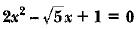has

Solution:

We have a quadratic equation:If we have standard equation ax2 + bx + c  then D = b2 - 4ac

a= 2, b= -√5, c= 1

D = (-√5)2 - (4x2x1)

D= 5 - 8

D = -3

As the value of D<0 so there is no real root

QUESTION: 6

Root of the equation x2 - 0.09 = 0 is

Solution:

x2 - 0.09 = 0
x2 = 0.09
x = √0.09
x = 0.3

QUESTION: 7

If 1/2  is a root of the equation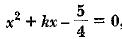then the value of k is

Solution:

As 1/2 is a root then it will satisfy the given equation.

Put x = 1/2

1/4 +(k×1/2) - 5/4 =0
k×1/2 =5/4 -1/4
k =2

QUESTION: 8

If the equation x2 - kx +9 = 0 does not possess real roots, then

Solution:
QUESTION: 9

Which of the following equations has - 1 as a root?

Solution:

3x² - 2x - 5 = 0
3x² + 3x - 5x - 5 = 0
3x(x+1) - 5(x+1) = 0
(x+1)(3x-5) = 0
x = -1 and 5/3.

Hence roots of given polynomial are -1 and 5/3.

QUESTION: 10

The quadratic equation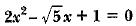has

Solution:

As, discriminant (b2 - 4ac) of the equation is negative. Therefore, no real roots.